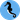# Hippocampus's Garden

Under the sea, in the hippocampus's garden...

# Meet Pandas: loc, iloc, at & iat

April 27, 2020  |  4 min read  |  318 views

Have you ever confused the Pandas methods loc, iloc, at, and iat with each other? Pandas is a great library for handling tabular data, but its API is too diverse and somewhat unintuitive. Understanding its design concepts might help it to some extent 🐼

So, this post aims to help understand differences between the Pandas methods loc, iloc, at, and iat. In short, the differences are summarized in the table below:

Single Multiple
label based at loc
integer position based iat iloc

* If this table makes sense, you won’t need this post any more.

Let’s find the differences using a simple example!

In this post, I use the iris dataset in the scikit-learn. The snippets in this post are supposed to be executed on Jupyter Notebook, Colaboratory, and stuff.

import pandas as pd

df = pd.DataFrame(iris.data, columns=iris.feature_names)
df

The dataframe should look something like this.

## at & iat Access a Scalar Value

If you just want to access a scalar value in the dataframe, it is fastest to use the methods at and iat. They both take two arguments to specify the row and the column to access, and produce the same outputs. The difference between them are discussed afterwards.

print(df.at[0, "sepal width (cm)"])
# 3.5
print(df.iat[0, 1])
# 3.5

## loc & iloc Access Multiple Values

When you want to access a scalar value, the methods loc and iloc are a bit slower but produce the same outputs as the methods at and iat.

print(df.loc[0, "sepal width (cm)"])
# 3.5
print(df.iloc[0, 1])
# 3.5

However, the methods loc and iloc can also access multiple values at a time. The following two statements give the same results: the values at the first row and the first two columns.

print(df.loc[0, :"sepal width (cm)"])
print(df.iloc[0, :2])
# sepal length (cm)    5.1
# sepal width (cm)     3.5
# Name: 0, dtype: float64 

The sliced form of the second argument is invalid for the methods at and iat.

print(df.at[0, :"sepal width (cm)"])
print(df.iat[0, :2])
# TypeError: unhashable type: 'slice'

You can input boolean arrays to specify rows and columns to access.

print(df.loc[0, [True, True, False, False]])
print(df.iloc[0, :2])
# sepal length (cm)    5.1
# sepal width (cm)     3.5
# Name: 0, dtype: float64

## at & loc vs. iat & iloc

So, what exactly is the difference between at and iat, or loc and iloc? I first thought that it’s the type of the second argument. Not accurate.

The methods at and loc access the values based on its labels, while the methods iat and iloc access the values based on its integer positions.

This difference is clear when you sort the dataframe.

df_sorted = df.sort_values("sepal width (cm)")
df_sorted

Note that the indices are re-ordered according to the sepal width (cm) column.

Now, the label-based df_sorted.at[0, "sepal width (cm)"] finds the row labeled 0 but the position-based df_sorted.iat[0, 1] finds the row at the top. The relationship between the methods loc and iloc is the same. Therefore:

print(df_sorted.at[0, "sepal width (cm)"])
# 3.5
print(df_sorted.iat[0, 1])
# 2.0
print(df_sorted.loc[0, "sepal width (cm)"])
# 3.5
print(df_sorted.iloc[0, 1])
# 2.0

## Conclusion

I hope this helps someone understand the differences between these confusing methods.

That’s it for today. Stay safe!Hippocampus's Garden © 2020, Shion Honda. Built with Gatsby